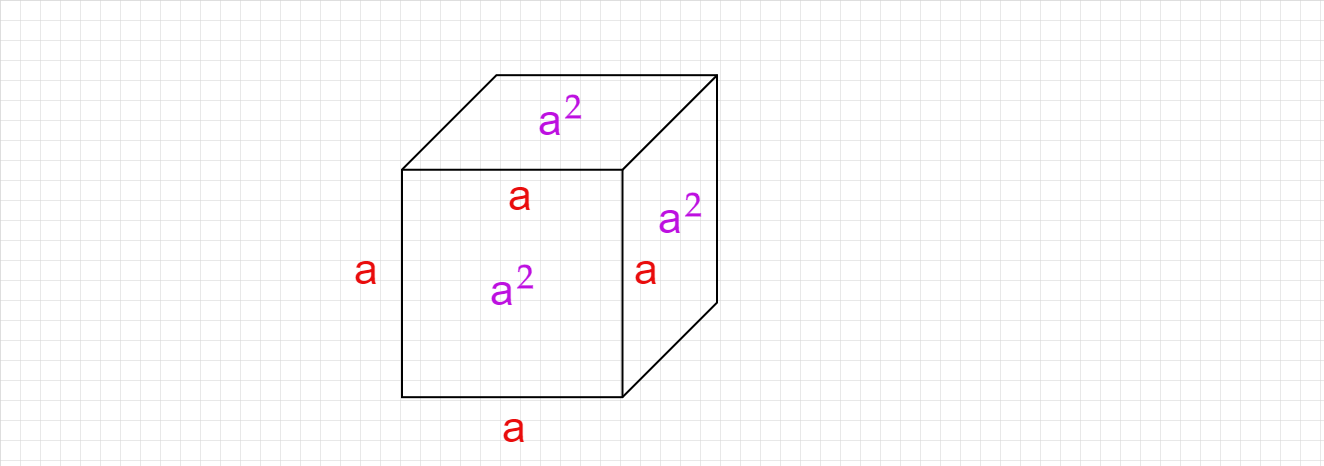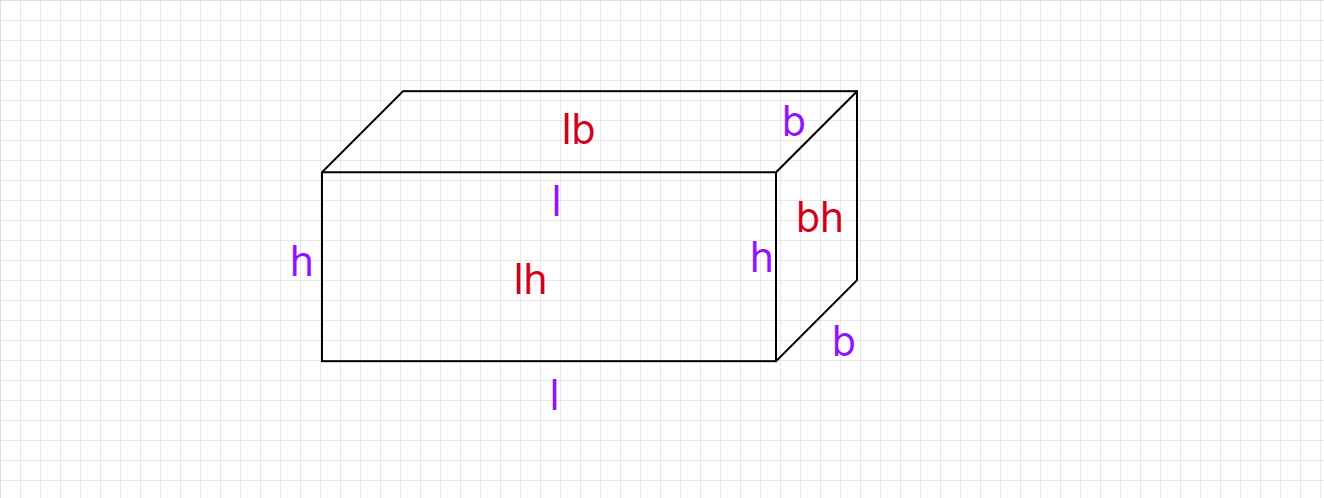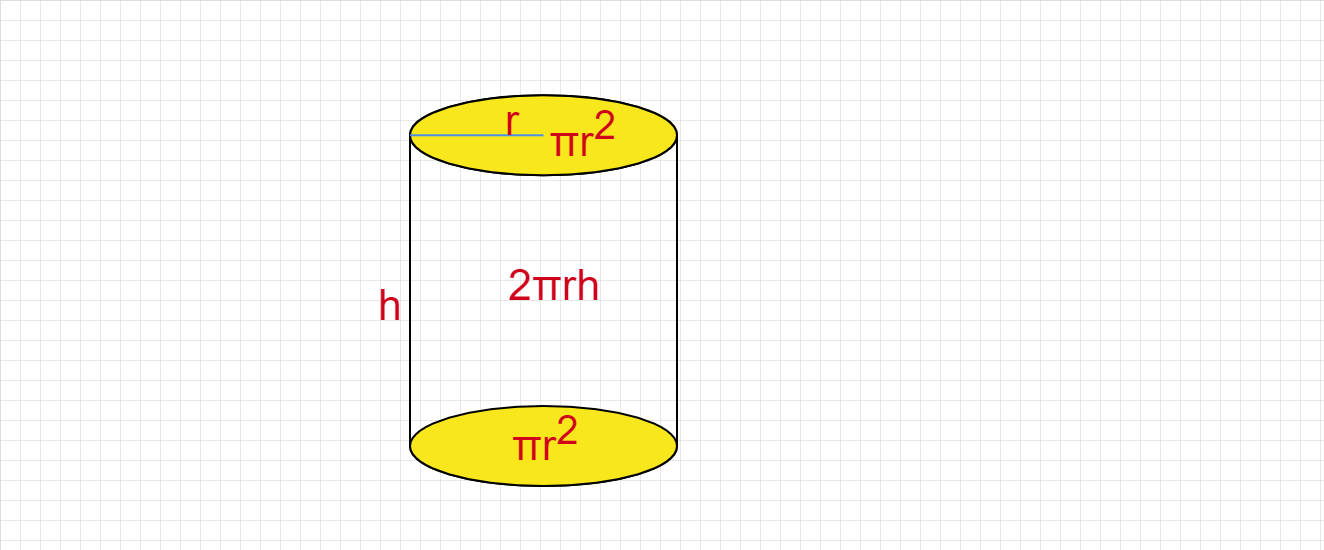# Explain the total surface areas of Cube, Cuboid, and Cylinder.

The total surface area of the Cube :

There are six square surfaces with side 'a' in the cube.

Therefore, the total surface area of cube $=6a^2$.The total surface area of the Cuboid :

The cuboid has six rectangular surfaces.

The surface area of cuboid with length 'l', breadth 'b', and height 'h' is given by,

Area of top and bottom surfaces $= lb+lb = 2lb$

Area of front and back surfaces $= lh+lh=2lh$

Area of surfaces in two sides $= bh+bh=2bh$

Total surface area $= 2 lb + 2 lh + 2 bh$

Total surface area of cuboid $= 2( lb + lh + bh)$The total surface area of a cylinder :

A cylinder has a curved surface and two circles at the top and the bottom surface.

The surface area of a cylinder with height 'h' and radius 'r' is given by,

Curved surface area $=2πrh$

Area of circles at the top and bottom surfaces $= πr^2 + πr^2= 2πr^2$

The total surface area $= 2πrh + 2πr^2 = 2πr(h+r)$

The total surface area of the cylinder $=2πr(h+r)$Updated on: 10-Oct-2022

62 Views